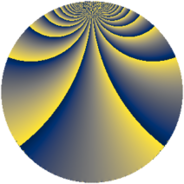# Properties

 Label 1216.4.bdLevel $1216$ Weight $4$ Character orbit 1216.bd Rep. character $\chi_{1216}(77,\cdot)$ Character field $\Q(\zeta_{16})$ Dimension $3456$ Sturm bound $640$

# Related objects

## Defining parameters

 Level: $$N$$ $$=$$ $$1216 = 2^{6} \cdot 19$$ Weight: $$k$$ $$=$$ $$4$$ Character orbit: $$[\chi]$$ $$=$$ 1216.bd (of order $$16$$ and degree $$8$$) Character conductor: $$\operatorname{cond}(\chi)$$ $$=$$ $$64$$ Character field: $$\Q(\zeta_{16})$$ Sturm bound: $$640$$

## Dimensions

The following table gives the dimensions of various subspaces of $$M_{4}(1216, [\chi])$$.

Total New Old
Modular forms 3856 3456 400
Cusp forms 3824 3456 368
Eisenstein series 32 0 32

## Trace form

 $$3456q + O(q^{10})$$ $$3456q - 944q^{22} - 2000q^{24} + 4640q^{30} + 1760q^{36} - 6320q^{42} - 2000q^{44} + 6624q^{52} - 1152q^{55} - 784q^{56} - 4752q^{58} + 5504q^{59} - 2928q^{62} + 10080q^{63} + 8160q^{67} - 2064q^{68} + 896q^{71} + 1296q^{72} + 5264q^{74} - 8832q^{75} + 3984q^{78} - 9280q^{80} - 13920q^{82} - 8288q^{84} + 1040q^{86} + 25232q^{92} + O(q^{100})$$

## Decomposition of $$S_{4}^{\mathrm{new}}(1216, [\chi])$$ into newform subspaces

The newforms in this space have not yet been added to the LMFDB.

## Decomposition of $$S_{4}^{\mathrm{old}}(1216, [\chi])$$ into lower level spaces

$$S_{4}^{\mathrm{old}}(1216, [\chi]) \cong$$ $$S_{4}^{\mathrm{new}}(64, [\chi])$$$$^{\oplus 2}$$